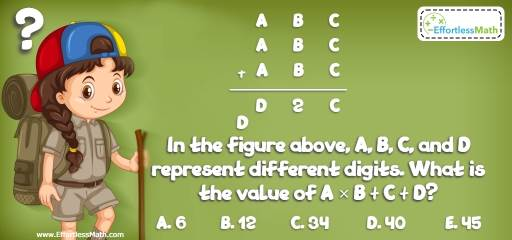# Intelligent Math Puzzle – Challenge 88

A great mathematical and logic puzzle designed for those interested in school-level Maths. The answer is also given.## Challenge:

In the figure above, A, B, C, and D represent different digits. What is the value of A × B + C + D?

A- 6

B- 12

C- 34

D- 40

E- 45

### The Absolute Best Book to challenge your Smart Student!

A, B, C, and D represent different digits. The sum of three 3-digit numbers is a 4-digit number with 1 or 2 in the thousands place. Therefore, D = 1 or 2. And A is 3 or greater than 3. Why?
A + A + A = 12 or 12+1, Why?
Since, A is a digit, then 3A cannot be 13. 3A = 12 ⇒ A = 4
We also know that C + C + C = C or C + 10
C = 0 or 3C = C + 10 → 2C = 10 → C = 5
Therefore, C is 0 or 5.
Let’s assume D = 1. Then:
If C = 0 → B + B + B = 1 or B + B + B = 1 + 10
3B = 1 (This is not correct) or 3B = 1 + 10 (This is not correct either).
If C = 5 → B + B + B + 1 = D → D = 1 → 3B = 1 – 1= 0
or
B + B + B + 1 = D + 10 → 3B + 1 = 1 + 10 → B $$= \frac{10}{3}$$
B is an integer and cannot be $$\frac{10}{3}$$.
In this situation, the only value of B is 0.
If A = 4, B = 0 and C = 5, then 405 + 405 + 405 = 1215 Bingo!
You can check out other values for A, B, C, and D. But, only the above values work.
A × B + C + D = 4 × 0 + 5 + 1 = 6

### What people say about "Intelligent Math Puzzle – Challenge 88 - Effortless Math: We Help Students Learn to LOVE Mathematics"?

No one replied yet.

X
30% OFF

Limited time only!

Save Over 30%

SAVE $5 It was$16.99 now it is \$11.99